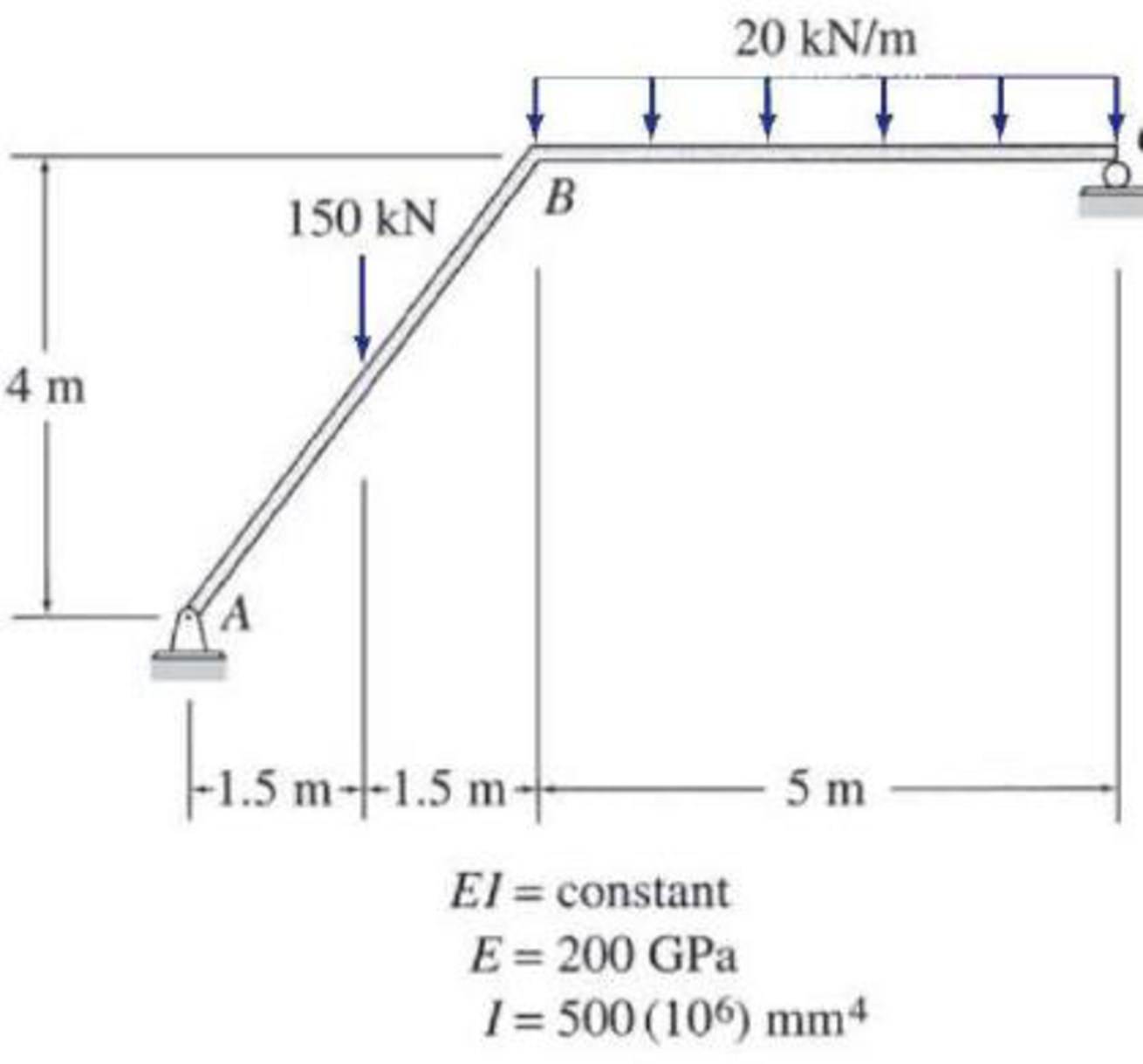# Use the virtual work method to determine the vertical deflection at joint B of the frame shown in Fig. P7.41.

#### Solutions

Chapter
Section
Chapter 7, Problem 42P
Textbook Problem
813 views

## Use the virtual work method to determine the vertical deflection at joint B of the frame shown in Fig. P7.41.To determine

Find the vertical deflection at joint B of the frame using virtual work method.

### Explanation of Solution

Given information:

The frame is given in the Figure.

The value of E is 200 GPa and I is 500×106mm4

Apply the sign conventions for calculating reactions, forces and moments using the three equations of equilibrium as shown below.

• For summation of forces along x-direction is equal to zero (Fx=0), consider the forces acting towards right side as positive (+) and the forces acting towards left side as negative ().
• For summation of forces along y-direction is equal to zero (Fy=0), consider the upward force as positive (+) and the downward force as negative ().
• For summation of moment about a joint is equal to zero (Matapoint=0), consider the clockwise moment as negative and the counter clockwise moment as positive.

Calculation:

Consider the real system.

Draw a diagram showing all the given real loads acting on it.

Let the bending moment due to real load be M.

Sketch the real system of the frame as shown in Figure 1.

Find the reactions at the supports:

Summation of moments about A is equal to 0.

MA=0Cy(8)20(5)(52+3)150(1.5)=0Cy=96.875kN

Summation of forces along y-direction is equal to 0.

+Fy=0Ay+Cy20(5)150=0Ay+96.87520(5)150=0Ay=153.125kN

The inclined horizontal reaction at A is,

=153.125×sin(9053.13°)=91.875kN

The inclined vertical reaction at A is,

=153.125×cos(9053.13°)=122.5kN

Consider the virtual system.

Draw a diagram of frame without the given real loads. For vertical deflection apply unit load at the joint in the vertical direction.

Let the bending moment due to virtual load be Mv.

Sketch the virtual system of the frame with unit load at joint B as shown in Figure 2.

Find the reactions at the supports:

Summation of moments about A is equal to 0.

MA=0Cy(8)1(3)=0Cy=0.375kN

Summation of forces along y-direction is equal to 0.

+Fy=0Ay+Cy1=0Ay+0.3751=0Ay=0.625kN

The inclined horizontal reaction at A is,

=0.625×sin(9053.13°)=0.375kN

The inclined vertical reaction at A is,

=0.625×cos(9053.13°)=0.5kN

Find the equations for M and Mv for the 3 segments of the frame as shown in Table 1.

 Segment x-coordinate M (kN⋅m) Mv (kN⋅m) Origin Limits (m) AD A 0−2.5 91.875x 0.375x DB A 2.5−5 1.875x+225 0.375x CD C 0−5 96.875x−10x2 0.375x

Find the vertical deflection at B using the virtual work expression:

ΔB=0LMvMEIdx (1)

Rearrange Equation (1) for the limits 02.5, 2.55, and 05 as follows.

ΔB=1EI[02

### Still sussing out bartleby?

Check out a sample textbook solution.

See a sample solution

#### The Solution to Your Study Problems

Bartleby provides explanations to thousands of textbook problems written by our experts, many with advanced degrees!

Get Started

Find more solutions based on key concepts
Why is it important to get to know upper-division engineering students?

Engineering Fundamentals: An Introduction to Engineering (MindTap Course List)

Convert 5 megawatts of power into BTU/hr, ft-lbs/s, and kJ/hr.

Fundamentals of Chemical Engineering Thermodynamics (MindTap Course List)

What is calibration and why is it important?

Precision Machining Technology (MindTap Course List)

Mobile Device Synchronization When you plug your smartphone into your computer to synchronize the data, the com...

Enhanced Discovering Computers 2017 (Shelly Cashman Series) (MindTap Course List)

Define an equipment grounding conductor.

EBK ELECTRICAL WIRING RESIDENTIAL

In what two ways can ultraviolet light burn the eyes?

Welding: Principles and Applications (MindTap Course List)

If your motherboard supports ECC DDR3 memory, can you substitute non-ECC DDR3 memory?

A+ Guide to Hardware (Standalone Book) (MindTap Course List)